PSABHXF - Elementary Algebra Accuplacer ASSISTments Evaluation - A
A 20% iodine solution is mixed with a 70% iodine solution to produce 5 gallons of 50% Iodine solution. How many gallons of each solution are needed?
Select one:

Graph the linear inequality. y<-3x+1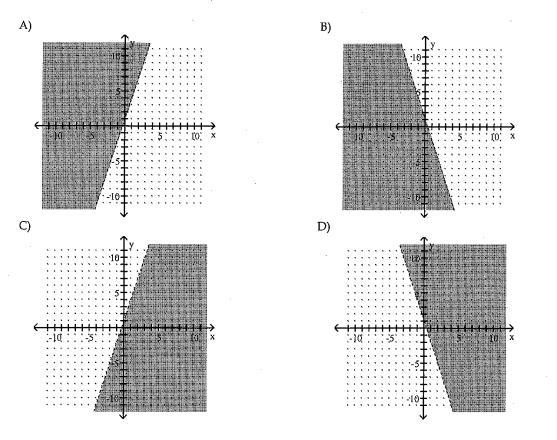Select one:

Factor: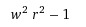Select one:
A car rental buisness rents a compact car at a daily rate of \$38.20 plus 20 cents per mile. Mike can afford to spend \$63 on the rental car for one day. How many miles can he drive and stay within his budget?
Select one:

Divide: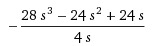Select one:

Simplify, assuming all variables represent non negative real numbers.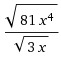Select one:
Simplify, assuming all variables represent non negative real numbers. sqrt(32yx^2)
Select one:
Determine whether 3 is a solution of the equation 9y + 8(y-2) = 35
Select one:

(x+9)(x-5) =  -13

Select one:
Simplify: -9 + 5(-2) - 11
Select one:
 Divide: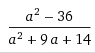by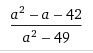Select one:
Multiply (4x - 1)(6x + 2)
Select one:

In the right triangle shown, find the length of the missing side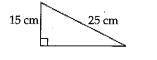Select one:

Solve the system of equations: 5x + y = 24 and x + 3y = 2

Select one: